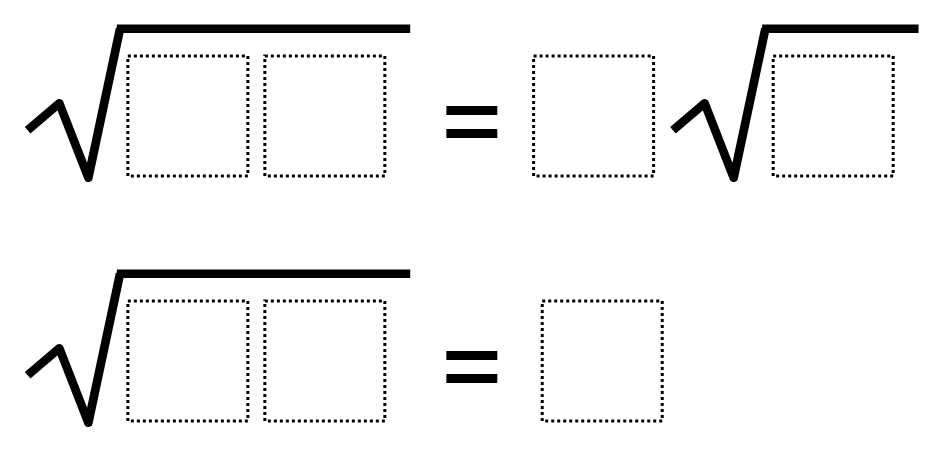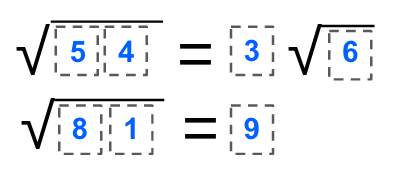Directions: Using the digits 0-9 at most one time each, make both of these equations true.### Hint

What are some two digit numbers that have a perfect square as a factor?

Multiple answers. Here is one possibility:Number of Unique Solutions: 8
1: sqrt(16) = 2sqrt(4) and sqrt(9) = 3
2: sqrt(18) = 3sqrt(2) and sqrt(49) = 7
3: sqrt(54) = 3sqrt(6) and sqrt(81) = 9
4: sqrt(64) = 8sqrt(1) and sqrt(9) = 3
5: sqrt(72) = 3sqrt(8) and sqrt(16) = 4
6: sqrt(81) = 3sqrt(9) and sqrt(4) = 2
7: sqrt(09) = 3sqrt(1) and sqrt(64) = 8
8: sqrt(98) = 7sqrt(2) and sqrt(16) = 4

Source: Jonathan Newman

## Polar and Cartesian form of complex numbers

Directions: Use the digits 1- 9, at most one time each, to fill in the …

1.I think there are only three solutions. The one in the example and

Sqrt(18) = 3*Sqrt(2) ; Sqrt(49) = 7

Sqrt(98) = 7*Sqrt(2) ; Sqrt(16) = 4

•2.